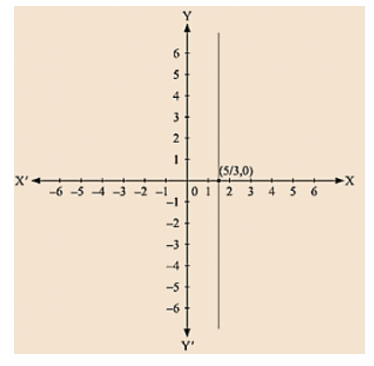# Give the geometric representations of the following equations

Question:

Give the geometric representations of the following equations

(a) on the number line (b) on the Cartesian plane:

(i) x = 2

(ii) y + 3 = 0

(iii) y = 3

(iv) 2x + 9 = 0

(v) 3x - 5 = 0

Solution:

(i) We are given, x = 2

The representation of the solution on the number line, when given equation is treated as an equation in one variable.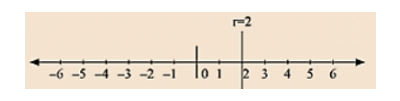The representation of the solution on the Cartesian plane, it is a line parallel to y axis passing through-the point (2, 0) is shown below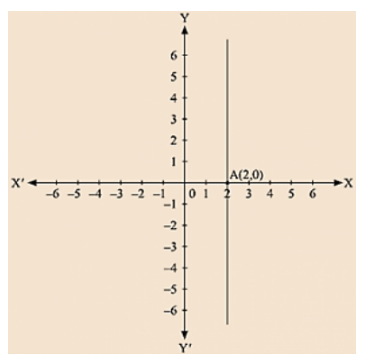(ii)  We are given, y + 3 = 0

We get, Y = – 3

The representation of the solution on the number line, when given equation is treated as an equation in one variable.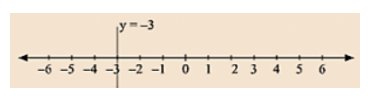The representation of the solution on the Cartesian plane, it is a line parallel to x axis passing through the point A(0, –3) is shown below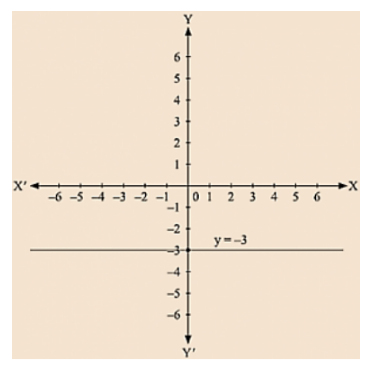(iii) we are given. y = 3

The representation of the solution on the number line. when given equation is treated as an equation in one variable.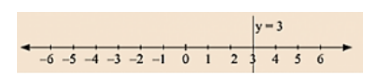The representation of the solution on the Cartesian plane, it is a line parallel to x axis passing through the point (0, 3) is shown below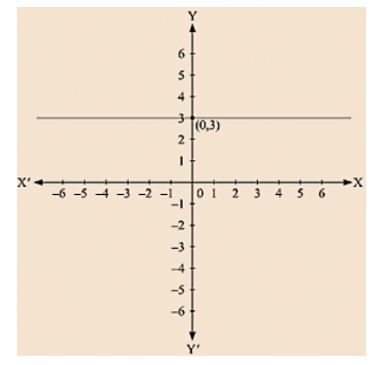(iv) We are given, 2x + 9 = 0

We get, 2x = - 9

The representation of the solution on the number line, when given equation is treated as an equation in one variable.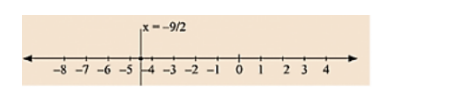The representation of the solution on the Cartesian plane, it is a line parallel to y axis passing through the point (-9/2, 0) is shown below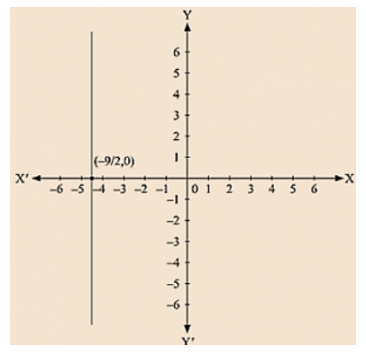(v) We are given, 3x - 5 = 0

We get, 3x = 5

The representation of the solution on the number line, when given equation is treated as an equation in one variable.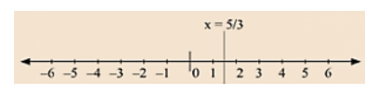The representation of the solution on the Cartesian plane, it is a line parallel to y axis passing through-the point (5, 0) is shown below